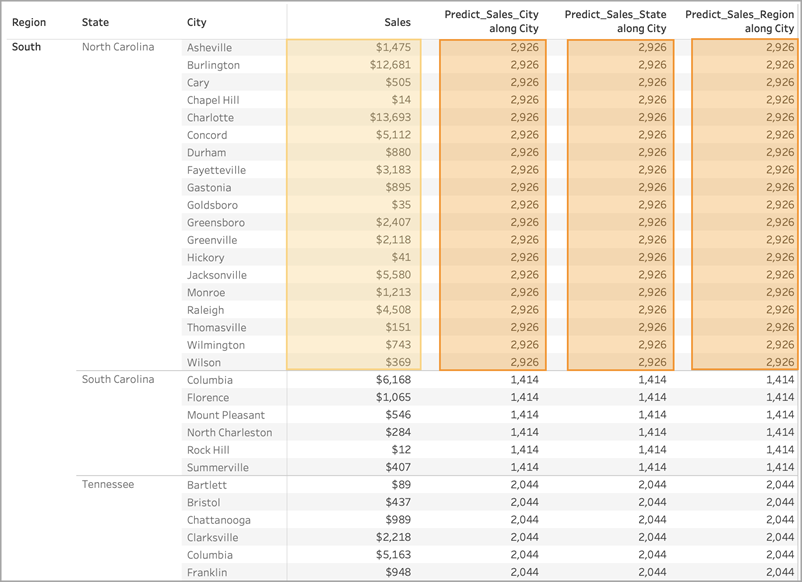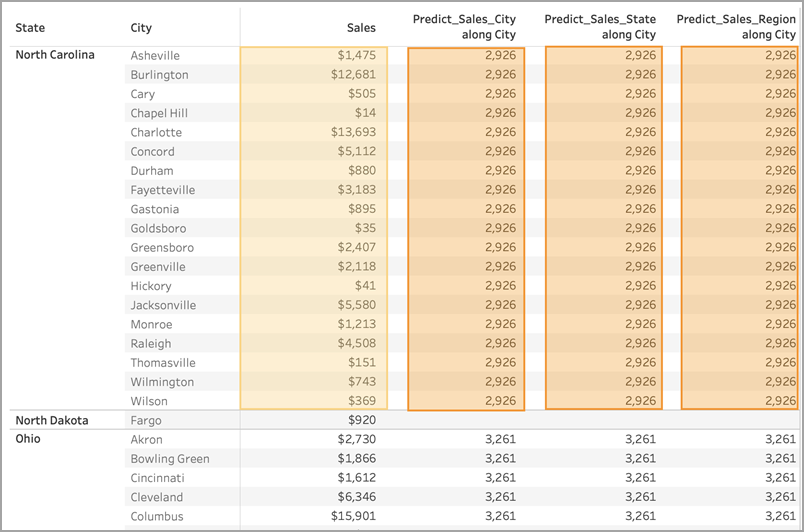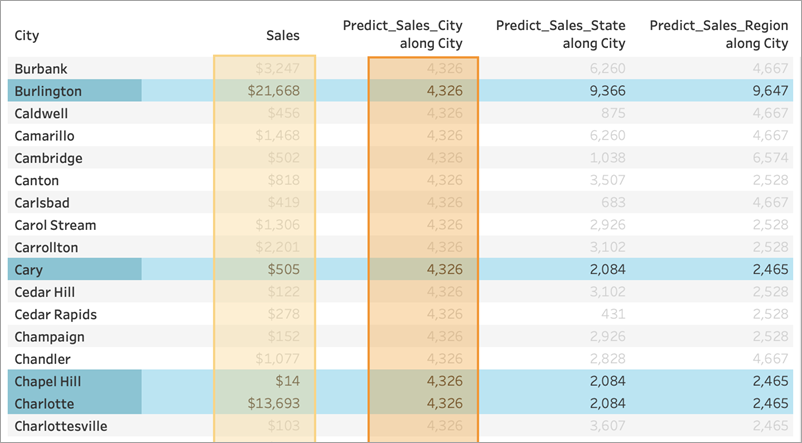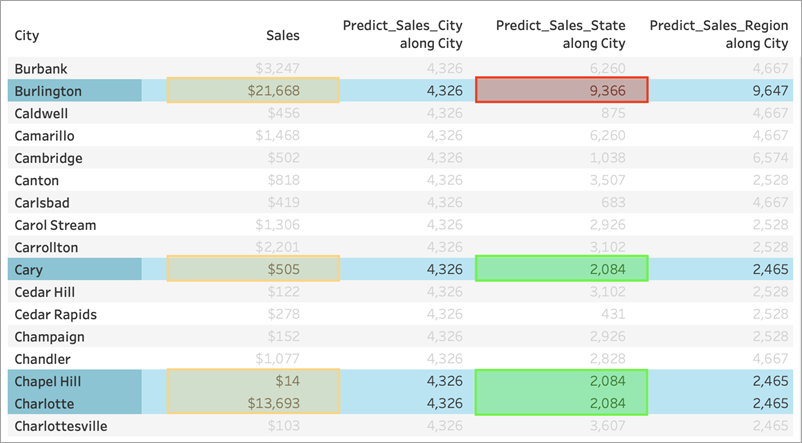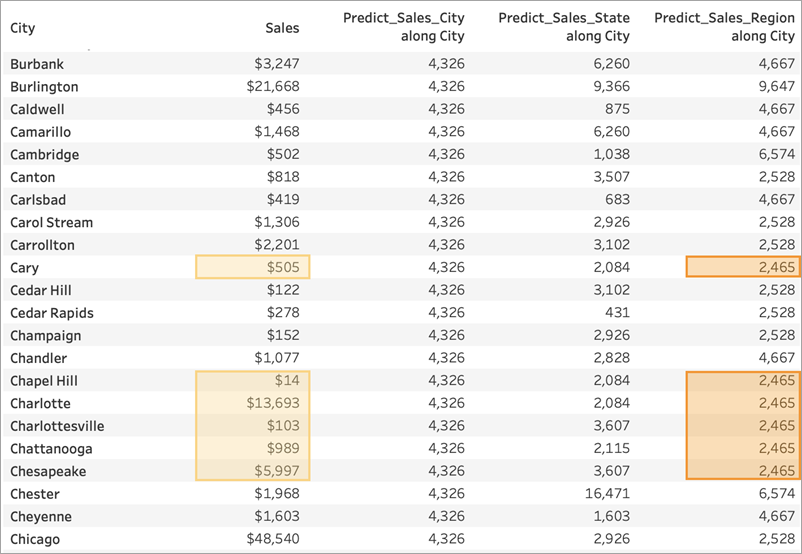# 预测建模中的计算依据和数据分区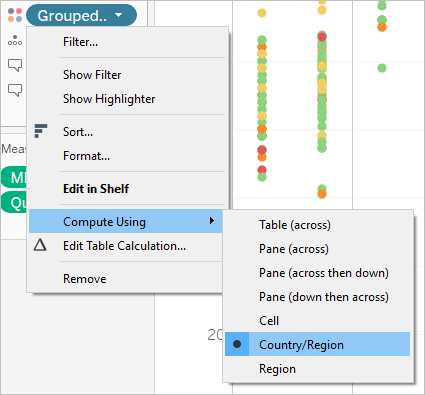## 针对预测建模函数的建议

### 选择维度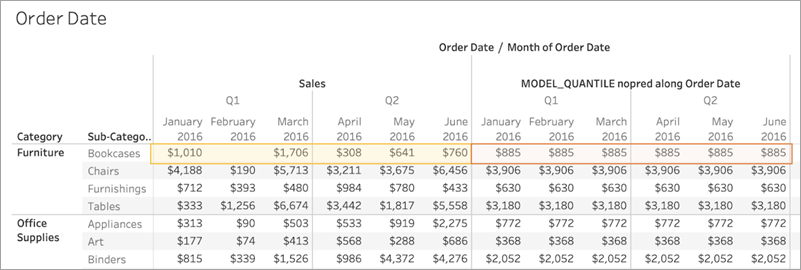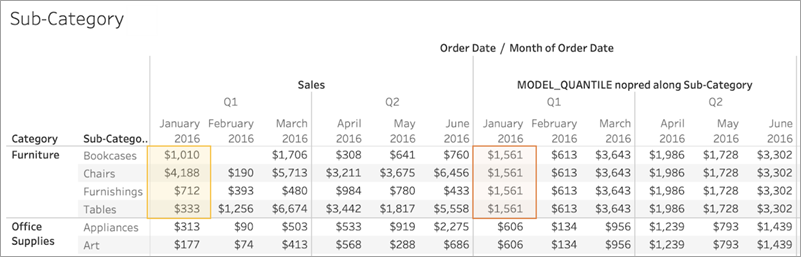## 关于分区的注意事项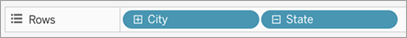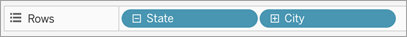Predict_Sales_City Predict_Sales_State Predict_Sales_Region ```MODEL_QUANTILE(0.5,sum([Sales]), ATTR([City]))``` ```MODEL_QUANTILE(0.5,sum([Sales]), ATTR([State]))``` ```MODEL_QUANTILE(0.5,sum([Sales]), ATTR([Region]))```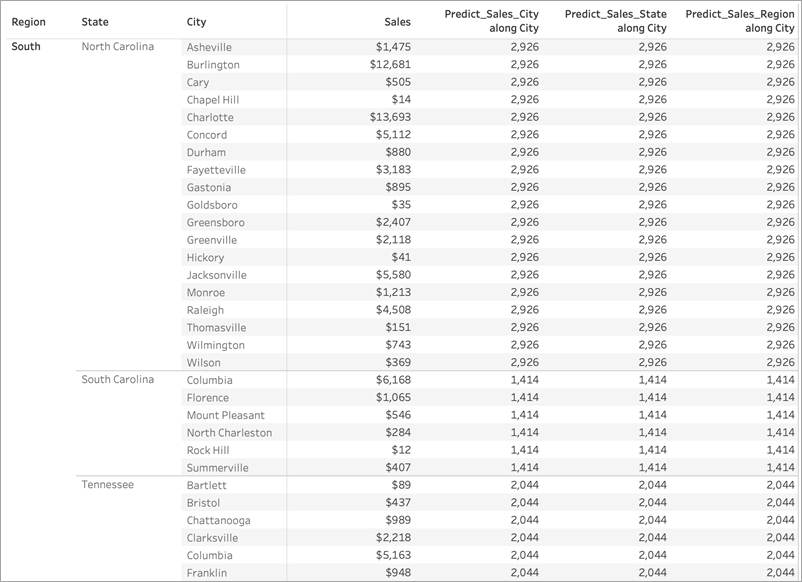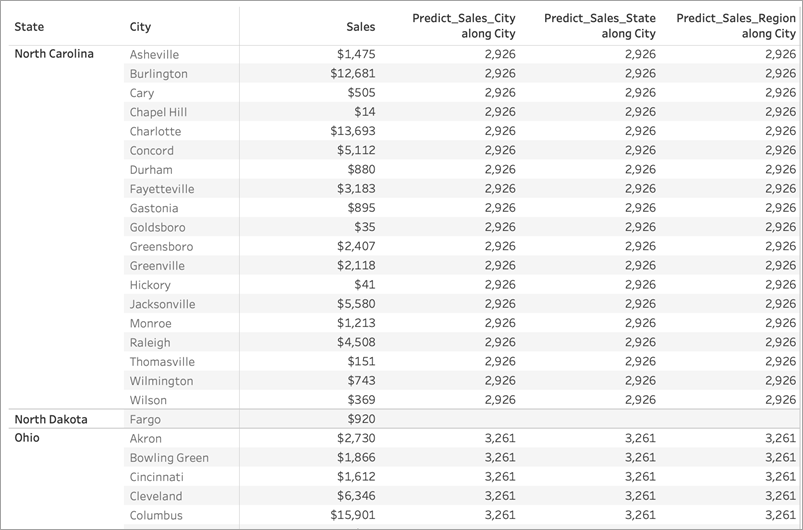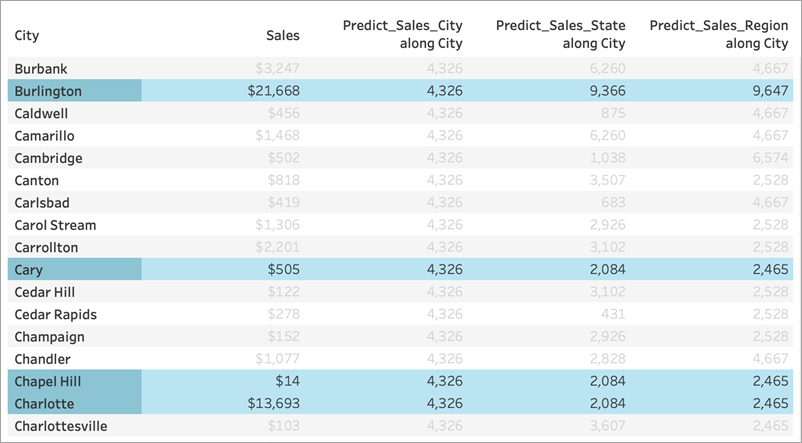### 怎么回事？